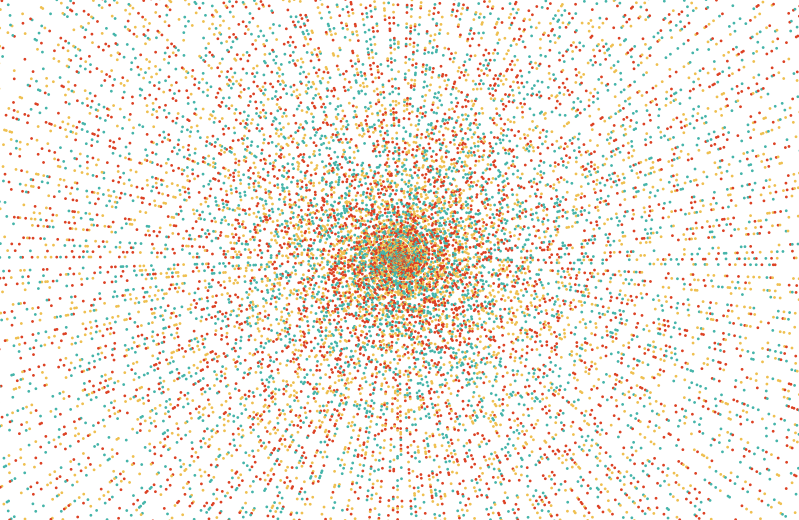# A Polar visualisation of prime numbers

Prime numbers exhibit an interesting structure. Projecting them onto a 2D plane results in:Projection of prime numbers on a 2D plane.

The image was generated with the following code:

import matplotlib.pyplot as plt
import numpy as np

def primes(n):
""" Returns  a list of primes < n """
sieve = [True] * n
for i in range(3,int(n**0.5)+1,2):
if sieve[i]:
sieve[i*i::2*i]=[False]*((n-i*i-1)//(2*i)+1)
return  + [i for i in range(3,n,2) if sieve[i]]

p = primes(5000000)
x = p * np.cos(p)
y = p * np.sin(p)

colors_possible = [[239, 192, 80], [221, 65, 36], [69, 181, 170]]
indices = np.random.randint(0,len(colors_possible),size=len(p))
colors = np.array([colors_possible[index] for index in indices])

plt.figure(figsize=(7,7))
axes = plt.gca()
axes.set_xlim([-100000,100000])
axes.set_ylim([-100000,100000])
plt.scatter(x,y, c=colors/255., s=1)
plt.show()

Written on October 25, 2020